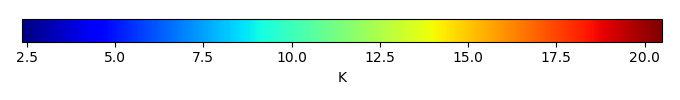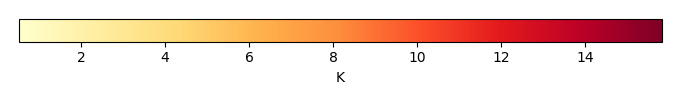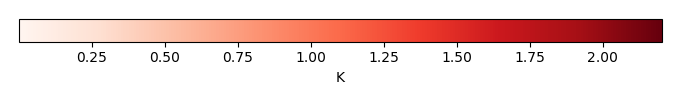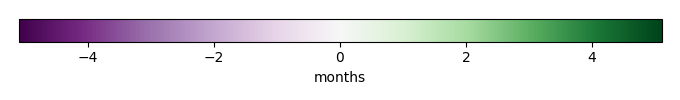# Mean State

Period Mean (original grids) [K]
Model Period Mean (intersection) [K]
Model Period Mean (complement) [K]
Benchmark Period Mean (intersection) [K]
Benchmark Period Mean (complement) [K]
Bias [K]
RMSE [K]
Phase Shift [months]
Bias Score 
RMSE Score 
Seasonal Cycle Score 
Spatial Distribution Score 
Interannual Variability Score 
Overall Score 
Benchmark [-] 10.8
CRUNCEPv7 [-] 8.70 8.73 5.31 10.9 8.01 -2.31 2.69 1.11 0.219 0.382 0.830 0.988 0.548 0.558
GSWP3v1 [-] 12.0 12.0 7.37 10.9 8.01 0.908 1.42 0.486 0.422 0.560 0.933 0.967 0.498 0.657
Period Mean (original grids) [K]
Model Period Mean (intersection) [K]
Model Period Mean (complement) [K]
Benchmark Period Mean (intersection) [K]
Benchmark Period Mean (complement) [K]
Bias [K]
RMSE [K]
Phase Shift [months]
Bias Score 
RMSE Score 
Seasonal Cycle Score 
Spatial Distribution Score 
Interannual Variability Score 
Overall Score 
Benchmark [-] 13.8
CRUNCEPv7 [-] 12.2 12.3 7.63 13.8 11.0 -1.51 3.34 0.949 0.539 0.444 0.903 0.850 0.548 0.621
GSWP3v1 [-] 14.7 14.8 9.12 13.8 11.0 0.904 1.34 0.512 0.715 0.736 0.947 0.991 0.777 0.817
Period Mean (original grids) [K]
Model Period Mean (intersection) [K]
Model Period Mean (complement) [K]
Benchmark Period Mean (intersection) [K]
Benchmark Period Mean (complement) [K]
Bias [K]
RMSE [K]
Phase Shift [months]
Bias Score 
RMSE Score 
Seasonal Cycle Score 
Spatial Distribution Score 
Interannual Variability Score 
Overall Score 
Benchmark [-] 12.8
CRUNCEPv7 [-] 11.3 11.4 5.41 12.8 9.07 -1.75 2.69 0.751 0.424 0.458 0.908 0.926 0.651 0.637
GSWP3v1 [-] 13.9 14.0 6.76 12.8 9.07 0.836 1.41 0.398 0.535 0.683 0.952 0.979 0.697 0.755
Period Mean (original grids) [K]
Model Period Mean (intersection) [K]
Model Period Mean (complement) [K]
Benchmark Period Mean (intersection) [K]
Benchmark Period Mean (complement) [K]
Bias [K]
RMSE [K]
Phase Shift [months]
Bias Score 
RMSE Score 
Seasonal Cycle Score 
Spatial Distribution Score 
Interannual Variability Score 
Overall Score 
Benchmark [-] 9.39
CRUNCEPv7 [-] 6.59 6.66 4.49 9.41 7.83 -2.95 3.53 0.910 0.247 0.382 0.895 0.924 0.777 0.601
GSWP3v1 [-] 9.65 9.75 6.02 9.41 7.83 0.0493 1.25 1.99 0.780 0.560 0.659 0.985 0.836 0.730
Period Mean (original grids) [K]
Model Period Mean (intersection) [K]
Model Period Mean (complement) [K]
Benchmark Period Mean (intersection) [K]
Benchmark Period Mean (complement) [K]
Bias [K]
RMSE [K]
Phase Shift [months]
Bias Score 
RMSE Score 
Seasonal Cycle Score 
Spatial Distribution Score 
Interannual Variability Score 
Overall Score 
Benchmark [-] 9.53
CRUNCEPv7 [-] 4.17 4.28 3.05 9.60 8.86 -5.66 5.70 1.54 0.00215 0.398 0.781 0.591 0.630 0.467
GSWP3v1 [-] 10.1 10.4 6.94 9.60 8.86 0.0612 1.72 0.907 0.226 0.493 0.875 0.963 0.765 0.636
Period Mean (original grids) [K]
Model Period Mean (intersection) [K]
Model Period Mean (complement) [K]
Benchmark Period Mean (intersection) [K]
Benchmark Period Mean (complement) [K]
Bias [K]
RMSE [K]
Phase Shift [months]
Bias Score 
RMSE Score 
Seasonal Cycle Score 
Spatial Distribution Score 
Interannual Variability Score 
Overall Score 
Benchmark [-] 10.7
CRUNCEPv7 [-] 8.34 8.36 4.32 10.7 8.60 -2.36 3.00 2.27 0.383 0.449 0.633 0.761 0.762 0.573
GSWP3v1 [-] 11.2 11.2 5.95 10.7 8.60 0.527 1.19 1.18 0.749 0.627 0.816 0.996 0.946 0.793
Period Mean (original grids) [K]
Model Period Mean (intersection) [K]
Model Period Mean (complement) [K]
Benchmark Period Mean (intersection) [K]
Benchmark Period Mean (complement) [K]
Bias [K]
RMSE [K]
Phase Shift [months]
Bias Score 
RMSE Score 
Seasonal Cycle Score 
Spatial Distribution Score 
Interannual Variability Score 
Overall Score 
Benchmark [-] 11.7
CRUNCEPv7 [-] 10.2 10.2 4.54 11.8 9.57 -1.73 2.92 1.92 0.419 0.384 0.699 0.991 0.812 0.615
GSWP3v1 [-] 12.1 12.1 6.61 11.8 9.57 0.152 1.04 0.675 0.748 0.642 0.907 0.998 0.917 0.809
Period Mean (original grids) [K]
Model Period Mean (intersection) [K]
Model Period Mean (complement) [K]
Benchmark Period Mean (intersection) [K]
Benchmark Period Mean (complement) [K]
Bias [K]
RMSE [K]
Phase Shift [months]
Bias Score 
RMSE Score 
Seasonal Cycle Score 
Spatial Distribution Score 
Interannual Variability Score 
Overall Score 
Benchmark [-] 11.1
CRUNCEPv7 [-] 10.2 10.4 4.37 11.2 8.50 -1.36 2.75 1.53 0.527 0.530 0.782 0.787 0.714 0.645
GSWP3v1 [-] 12.1 12.3 6.72 11.2 8.50 0.630 1.33 0.485 0.652 0.726 0.941 0.966 0.841 0.809
Period Mean (original grids) [K]
Model Period Mean (intersection) [K]
Model Period Mean (complement) [K]
Benchmark Period Mean (intersection) [K]
Benchmark Period Mean (complement) [K]
Bias [K]
RMSE [K]
Phase Shift [months]
Bias Score 
RMSE Score 
Seasonal Cycle Score 
Spatial Distribution Score 
Interannual Variability Score 
Overall Score 
Benchmark [-] 8.50
CRUNCEPv7 [-] 6.03 6.09 4.36 8.51 7.45 -2.52 3.12 1.83 0.305 0.398 0.700 0.961 0.744 0.584
GSWP3v1 [-] 8.46 8.53 5.66 8.51 7.45 -0.103 1.10 0.802 0.814 0.598 0.875 0.973 0.803 0.777
Period Mean (original grids) [K]
Model Period Mean (intersection) [K]
Model Period Mean (complement) [K]
Benchmark Period Mean (intersection) [K]
Benchmark Period Mean (complement) [K]
Bias [K]
RMSE [K]
Phase Shift [months]
Bias Score 
RMSE Score 
Seasonal Cycle Score 
Spatial Distribution Score 
Interannual Variability Score 
Overall Score 
Benchmark [-] 13.7
CRUNCEPv7 [-] 11.8 12.0 4.87 13.8 10.4 -2.33 3.14 0.841 0.412 0.477 0.920 0.691 0.802 0.630
GSWP3v1 [-] 14.7 14.8 7.22 13.8 10.4 0.576 1.27 0.383 0.601 0.699 0.964 0.920 0.909 0.799
Period Mean (original grids) [K]
Model Period Mean (intersection) [K]
Model Period Mean (complement) [K]
Benchmark Period Mean (intersection) [K]
Benchmark Period Mean (complement) [K]
Bias [K]
RMSE [K]
Phase Shift [months]
Bias Score 
RMSE Score 
Seasonal Cycle Score 
Spatial Distribution Score 
Interannual Variability Score 
Overall Score 
Benchmark [-] 8.57
CRUNCEPv7 [-] 6.69 6.75 4.82 8.60 7.69 -2.12 2.54 1.08 0.402 0.574 0.863 0.982 0.737 0.689
GSWP3v1 [-] 9.24 9.34 6.50 8.60 7.69 0.366 1.24 0.597 0.674 0.663 0.925 0.952 0.873 0.792
Period Mean (original grids) [K]
Model Period Mean (intersection) [K]
Model Period Mean (complement) [K]
Benchmark Period Mean (intersection) [K]
Benchmark Period Mean (complement) [K]
Bias [K]
RMSE [K]
Phase Shift [months]
Bias Score 
RMSE Score 
Seasonal Cycle Score 
Spatial Distribution Score 
Interannual Variability Score 
Overall Score 
Benchmark [-] 11.6
CRUNCEPv7 [-] 9.55 9.97 6.07 11.8 7.98 -2.11 3.26 1.35 0.340 0.423 0.806 0.958 0.687 0.606
GSWP3v1 [-] 12.0 12.5 7.83 11.8 7.98 0.418 1.27 0.642 0.634 0.643 0.912 0.993 0.799 0.770
Period Mean (original grids) [K]
Model Period Mean (intersection) [K]
Model Period Mean (complement) [K]
Benchmark Period Mean (intersection) [K]
Benchmark Period Mean (complement) [K]
Bias [K]
RMSE [K]
Phase Shift [months]
Bias Score 
RMSE Score 
Seasonal Cycle Score 
Spatial Distribution Score 
Interannual Variability Score 
Overall Score 
Benchmark [-] 14.0
CRUNCEPv7 [-] 15.1 15.2 8.63 14.0 11.1 0.874 2.91 1.21 0.374 0.325 0.837 0.823 0.626 0.552
GSWP3v1 [-] 15.3 15.3 8.54 14.0 11.1 1.05 1.55 0.520 0.452 0.620 0.932 0.967 0.732 0.720
Period Mean (original grids) [K]
Model Period Mean (intersection) [K]
Model Period Mean (complement) [K]
Benchmark Period Mean (intersection) [K]
Benchmark Period Mean (complement) [K]
Bias [K]
RMSE [K]
Phase Shift [months]
Bias Score 
RMSE Score 
Seasonal Cycle Score 
Spatial Distribution Score 
Interannual Variability Score 
Overall Score 
Benchmark [-] 8.40
CRUNCEPv7 [-] 6.49 6.55 4.79 8.41 7.44 -2.05 2.88 0.718 0.353 0.343 0.912 0.914 0.790 0.609
GSWP3v1 [-] 8.43 8.51 5.79 8.41 7.44 -0.161 1.04 0.718 0.811 0.582 0.890 0.986 0.904 0.792
Period Mean (original grids) [K]
Model Period Mean (intersection) [K]
Model Period Mean (complement) [K]
Benchmark Period Mean (intersection) [K]
Benchmark Period Mean (complement) [K]
Bias [K]
RMSE [K]
Phase Shift [months]
Bias Score 
RMSE Score 
Seasonal Cycle Score 
Spatial Distribution Score 
Interannual Variability Score 
Overall Score 
Benchmark [-] 13.3
CRUNCEPv7 [-] 8.73 8.84 6.00 13.4 10.3 -5.30 5.65 1.14 0.125 0.439 0.854 0.903 0.627 0.565
GSWP3v1 [-] 14.0 14.3 8.92 13.4 10.3 0.0148 1.56 0.524 0.568 0.617 0.938 0.968 0.799 0.751
Period Mean (original grids) [K]
Model Period Mean (intersection) [K]
Model Period Mean (complement) [K]
Benchmark Period Mean (intersection) [K]
Benchmark Period Mean (complement) [K]
Bias [K]
RMSE [K]
Phase Shift [months]
Bias Score 
RMSE Score 
Seasonal Cycle Score 
Spatial Distribution Score 
Interannual Variability Score 
Overall Score 
Benchmark [-] 12.2
CRUNCEPv7 [-] 8.51 8.51 5.16 12.2 9.97 -3.82 4.05 2.49 0.126 0.465 0.600 0.926 0.682 0.544
GSWP3v1 [-] 12.2 12.2 9.12 12.2 9.97 -0.132 1.01 0.920 0.735 0.640 0.856 0.903 0.939 0.785
Period Mean (original grids) [K]
Model Period Mean (intersection) [K]
Model Period Mean (complement) [K]
Benchmark Period Mean (intersection) [K]
Benchmark Period Mean (complement) [K]
Bias [K]
RMSE [K]
Phase Shift [months]
Bias Score 
RMSE Score 
Seasonal Cycle Score 
Spatial Distribution Score 
Interannual Variability Score 
Overall Score 
Benchmark [-] 11.0
CRUNCEPv7 [-] 8.46 8.47 4.62 11.1 7.54 -2.76 3.19 2.18 0.292 0.493 0.634 0.994 0.710 0.603
GSWP3v1 [-] 11.2 11.2 6.99 11.1 7.54 -0.00688 1.05 0.747 0.789 0.658 0.893 0.997 0.943 0.823
Period Mean (original grids) [K]
Model Period Mean (intersection) [K]
Model Period Mean (complement) [K]
Benchmark Period Mean (intersection) [K]
Benchmark Period Mean (complement) [K]
Bias [K]
RMSE [K]
Phase Shift [months]
Bias Score 
RMSE Score 
Seasonal Cycle Score 
Spatial Distribution Score 
Interannual Variability Score 
Overall Score 
Benchmark [-] 15.3
CRUNCEPv7 [-] 12.6 12.5 8.15 15.3 11.3 -2.87 3.81 0.798 0.353 0.393 0.920 0.991 0.848 0.650
GSWP3v1 [-] 15.1 15.1 9.45 15.3 11.3 -0.347 1.25 0.419 0.755 0.656 0.950 0.998 0.925 0.824
Period Mean (original grids) [K]
Model Period Mean (intersection) [K]
Model Period Mean (complement) [K]
Benchmark Period Mean (intersection) [K]
Benchmark Period Mean (complement) [K]
Bias [K]
RMSE [K]
Phase Shift [months]
Bias Score 
RMSE Score 
Seasonal Cycle Score 
Spatial Distribution Score 
Interannual Variability Score 
Overall Score 
Benchmark [-] 10.2
CRUNCEPv7 [-] 7.34 7.35 3.84 10.2 9.50 -2.99 3.17 0.604 0.146 0.507 0.919 0.985 0.456 0.587
GSWP3v1 [-] 11.1 11.1 6.95 10.2 9.50 0.766 1.11 0.435 0.501 0.657 0.947 0.989 0.466 0.703
Period Mean (original grids) [K]
Model Period Mean (intersection) [K]
Model Period Mean (complement) [K]
Benchmark Period Mean (intersection) [K]
Benchmark Period Mean (complement) [K]
Bias [K]
RMSE [K]
Phase Shift [months]
Bias Score 
RMSE Score 
Seasonal Cycle Score 
Spatial Distribution Score 
Interannual Variability Score 
Overall Score 
Benchmark [-] 11.7
CRUNCEPv7 [-] 9.20 9.29 4.36 11.7 9.16 -2.56 3.08 0.831 0.248 0.398 0.890 0.879 0.548 0.560
GSWP3v1 [-] 12.3 12.4 6.98 11.7 9.16 0.582 0.993 0.368 0.624 0.682 0.956 0.986 0.617 0.758
Period Mean (original grids) [K]
Model Period Mean (intersection) [K]
Model Period Mean (complement) [K]
Benchmark Period Mean (intersection) [K]
Benchmark Period Mean (complement) [K]
Bias [K]
RMSE [K]
Phase Shift [months]
Bias Score 
RMSE Score 
Seasonal Cycle Score 
Spatial Distribution Score 
Interannual Variability Score 
Overall Score 
Benchmark [-] 12.5
CRUNCEPv7 [-] 9.75 9.76 6.59 12.6 7.59 -3.28 3.85 1.36 0.218 0.473 0.815 0.988 0.668 0.606
GSWP3v1 [-] 13.5 13.5 8.56 12.6 7.59 0.279 1.39 0.801 0.622 0.612 0.901 0.978 0.735 0.743

# Temporally integrated period mean

BENCHMARK MEANMODEL MEANBIASBIAS SCORERMSERMSE SCOREBENCHMARK INTERANNUAL VARIABILITYMODEL INTERANNUAL VARIABILITYINTERANNUAL VARIABILITY SCOREBENCHMARK MAX MONTHMODEL MAX MONTHDIFFERENCE IN MAX MONTHSEASONAL CYCLE SCORESPATIAL TAYLOR DIAGRAMMODEL COLORS# Spatially integrated regional mean

MODEL COLORSREGIONAL MEANANNUAL CYCLEMONTHLY ANOMALYANNUAL CYCLE# All Models

BenchmarkCRUNCEPv7GSWP3v1# Data Information

creation_date: Fri Feb 8 13:55:45 PST 2019

history: 2018-01-15: download from https://crudata.uea.ac.uk/cru/data/hrg/cru_ts_4.01/cruts.1709081022.v4.01/ 2019-02-06: converted and created to netCDF4 for ILAMB package

original_resolution: 0.5x0.5

resolution: 0.5x0.5

original_frequency: mon

frequency: mon

references: Harris et al., 2014, Int. J. Climatol., doi:10.1002/joc.3711

institution: Original Data held at British Atmospheric Data Centre, RAL, UK.

source: Run ID = 1709081022. Data generated from:dtr.1708250000.dtb

title: CRU TS4.01 Diurnal Temperature Range

Conventions: CF-1.7